# Logarithmic spiral

(diff) ← Older revision | Latest revision (diff) | Newer revision → (diff)

A plane transcendental curve whose equation in polar coordinates has the formFigure: l060650a

If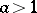, as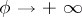the logarithmic spiral evolves anti-clockwise, and as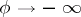the spiral twists clockwise, tending to its asymptotic point(see Fig.). If, the twisting behaviour is opposite. The angle formed by the tangent at an arbitrary point of the logarithmic spiral and the position vector of that point depends only on the parameter. The length of the arc between two points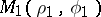andis: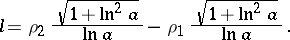The radius of curvature is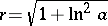. The natural equation is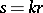, where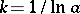. Logarithmic spirals go into logarithmic spirals under linear isometries, similarities and inversions of the plane. The logarithmic spiral is related to the so-called pseudo-spirals (see Spirals).

How to Cite This Entry:
Logarithmic spiral. Encyclopedia of Mathematics. URL: http://encyclopediaofmath.org/index.php?title=Logarithmic_spiral&oldid=17772
This article was adapted from an original article by D.D. Sokolov (originator), which appeared in Encyclopedia of Mathematics - ISBN 1402006098. See original article